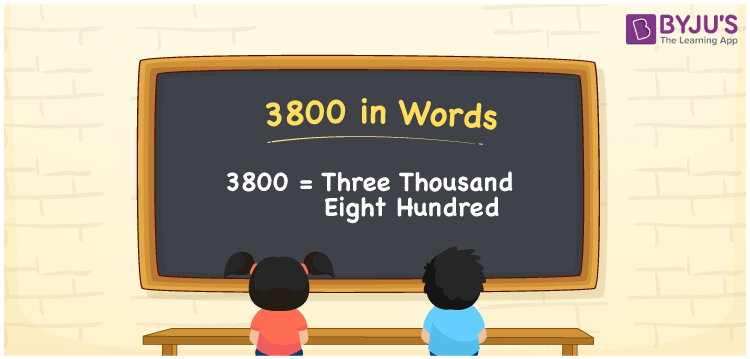# 3800 in Words

3800 in words can be written as Three Thousand Eight Hundred. Students will be able to learn the conversion of 3800 in words which will help them understand the applications of numbers in our daily lives. If you buy an earphone for Rs. 3800, then you can say that “I have bought an earphone for Three Thousand Eight Hundred Rupees”. The  number 3800 can be written in words using the English alphabet. The numbers in words can be grasped easily by the students using the resources given at BYJU’S. 3800 in English can be read as “Three Thousand Eight Hundred”.

 3800 in words Three Thousand Eight Hundred Three Thousand Eight Hundred in Numbers 3800

## 3800 in English Words## How to Write 3800 in Words?

Students will learn about the conversion of 3800 into words from place value charts. The number 3800 has four digits. For 3800, the place value chart is prepared in a table form to help students understand it effectively.

 Thousands Hundreds Tens Ones 3 8 0 0

3800 in expanded form is explained in brief here:

3 × Thousand + 8 × Hundred + 0 × Ten + 0 × One

= 3 × 1000  + 8 × 100 + 0 × 10 + 0 × 1

= 3000 + 800

= 3800

= Three Thousand Eight Hundred

Therefore, 3800 in words is written as Three Thousand Eight Hundred.

3800 is a natural number that precedes 3801 and succeeds 3799.

3800 in words – Three Thousand Eight Hundred

Is 3800 an odd number? – No

Is 3800 an even number? – Yes

Is 3800 a perfect square number? – Yes

Is 3800 a perfect cube number? – No

Is 3800 a prime number? – No

Is 3800 a composite number? – Yes

## Frequently Asked Questions on 3800 in Words

Q1

### How do you write 3800 in words?

3800 can be written as “Three Thousand Eight Hundred” in words.
Q2

### Is 3800 an even number?

3800 is an even number because it is divisible by 2.
3800/2 = 1900
Q3

### How can Three Thousand Eight Hundred be written in numbers?

Three Thousand Eight Hundred can be written in numbers as 3800.